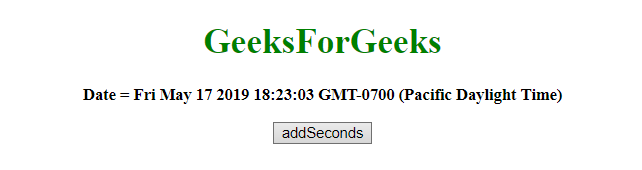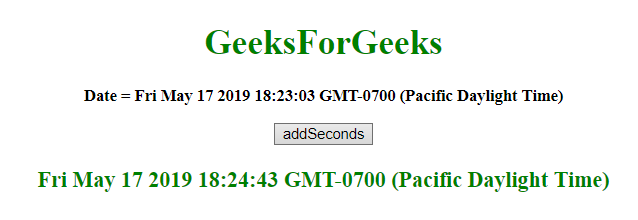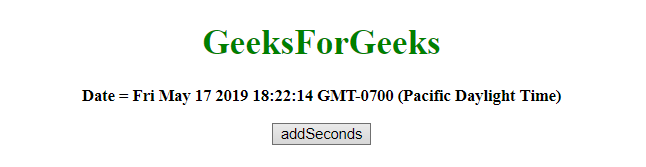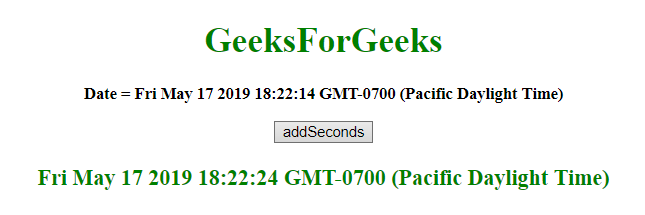Related Articles

# JavaScript | Adding seconds to Date object

• Last Updated : 23 May, 2019

Given a date, the task is to add seconds to it. To add seconds to date in javascript, we’re going to discuss a few techniques. First few methods to know.

• JavaScript getSeconds() Method
This method returns the Seconds(from 0 to 59) of the provided date and time.
Syntax:

Hey geek! The constant emerging technologies in the world of web development always keeps the excitement for this subject through the roof. But before you tackle the big projects, we suggest you start by learning the basics. Kickstart your web development journey by learning JS concepts with our JavaScript Course. Now at it's lowest price ever!

```Date.getSeconds()
```

Return value:
It returns a number, from 0 to 59, representing the seconds.

• JavaScript setSeconds() Method
This method set the seconds of a date object.
This method can also be used to set the milliseconds.
Syntax:

```Date.setSeconds(sec, millisec)
```

Parameters:

• sec: This parameter is optional. It specifies the integer representing the seconds. Values expected are 0-59, but other values are allowed.
• millisec: This parameter is optional. It specifies the integer representing the milliseconds. Values expected are 0-999, but other values are allowed.

Note:
All the previous 2 parameters accept values apart from their range and these values adjust like.

• sec = -1, means the last second of the previous minute and same for the other parameters.
• if sec passed is 60, means the first second of the next minute and same for the other parameters.

Return value:
It returns a number, denoting the number of milliseconds between the date object and midnight January 1, 1970.

• JavaScript getTime() method
This method returns the number of milliseconds between midnight of January 1, 1970, and the specified date.
Syntax:
```Date.getTime()
```

Return value:
It returns a number, representing the number of milliseconds since midnight January 1, 1970.

• JavaScript setTime() method
This method set the date and time by adding/subtracting a defined number of milliseconds to/from midnight January 1, 1970.
Syntax:

```Date.setTime(millisec)
```

Parameters:

• millisec: This parameter is required. It specifies the number of milliseconds to be added/subtracted, midnight January 1, 1970

Return value:
It returns, representing the number of milliseconds between the date object and midnight January 1, 1970.

Example 1: This example adds 100 seconds to the var today by using setTime() and getTime() method.

 ```<``html``>`` ` `<``head``>``    ``<``title``>``        ``JavaScript ``      ``| Adding Seconds to Date object.``    `````` ` `<``body` `style``=``"text-align:center;"` `      ``id``=``"body"``>``    ``<``h1` `style``=``"color:green;"``>  ``            ``GeeksForGeeks  ``        ````    ``<``p` `id``=``"GFG_UP"` `       ``style="font-size: 15px;``              ``font-weight: bold;">``    ````    ``<``button` `onclick``=``"gfg_Run()"``>``        ``addSeconds``    ````    ``<``p` `id``=``"GFG_DOWN"` `       ``style="color:green; ``              ``font-size: 20px; ``              ``font-weight: bold;">``    ````    ``<``script``>``        ``var el_up = ``            ``document.getElementById("GFG_UP");``        ``var el_down = ``            ``document.getElementById("GFG_DOWN");``        ``var today = new Date();``        ``el_up.innerHTML = "Date = " + today;``        ``Date.prototype.addSecs = function(s) {``            ``this.setTime(this.getTime() + (s * 1000));``            ``return this;``        ``}`` ` `        ``function gfg_Run() {``            ``var a = new Date();``            ``a.addSecs(100);``            ``el_down.innerHTML = a;``        ``}``    `````` ` ``

Output:

• Before clicking on the button:• After clicking on the button:Example 2: This example adds 10 seconds to the var today by using setSeconds() and getSeconds() method.

 ```<``html``>`` ` `<``head``>``    ``<``title``>``        ``JavaScript ``      ``| Adding Seconds to Date object.``    `````` ` `<``body` `style``=``"text-align:center;"` `      ``id``=``"body"``>``    ``<``h1` `style``=``"color:green;"``>  ``            ``GeeksForGeeks  ``        ````    ``<``p` `id``=``"GFG_UP"``       ``style="font-size: 15px;``              ``font-weight: bold;">``    ````    ``<``button` `onclick``=``"gfg_Run()"``>``        ``addSeconds``    ````    ``<``p` `id``=``"GFG_DOWN"` `       ``style="color:green; ``              ``font-size: 20px; ``              ``font-weight: bold;">``    ````    ``<``script``>``        ``var el_up = ``            ``document.getElementById("GFG_UP");``        ``var el_down =``            ``document.getElementById("GFG_DOWN");``        ``var today = new Date();``        ``el_up.innerHTML = "Date = " + today;``        ``Date.prototype.addSecs = function(s) {``            ``this.setSeconds(this.getSeconds() + s);``            ``return this;``        ``}`` ` `        ``function gfg_Run() {``            ``var a = new Date();``            ``a.addSecs(10);``            ``el_down.innerHTML = a;``        ``}``    `````` ` ``

Output:

• Before clicking on the button:• After clicking on the button:My Personal Notes arrow_drop_up